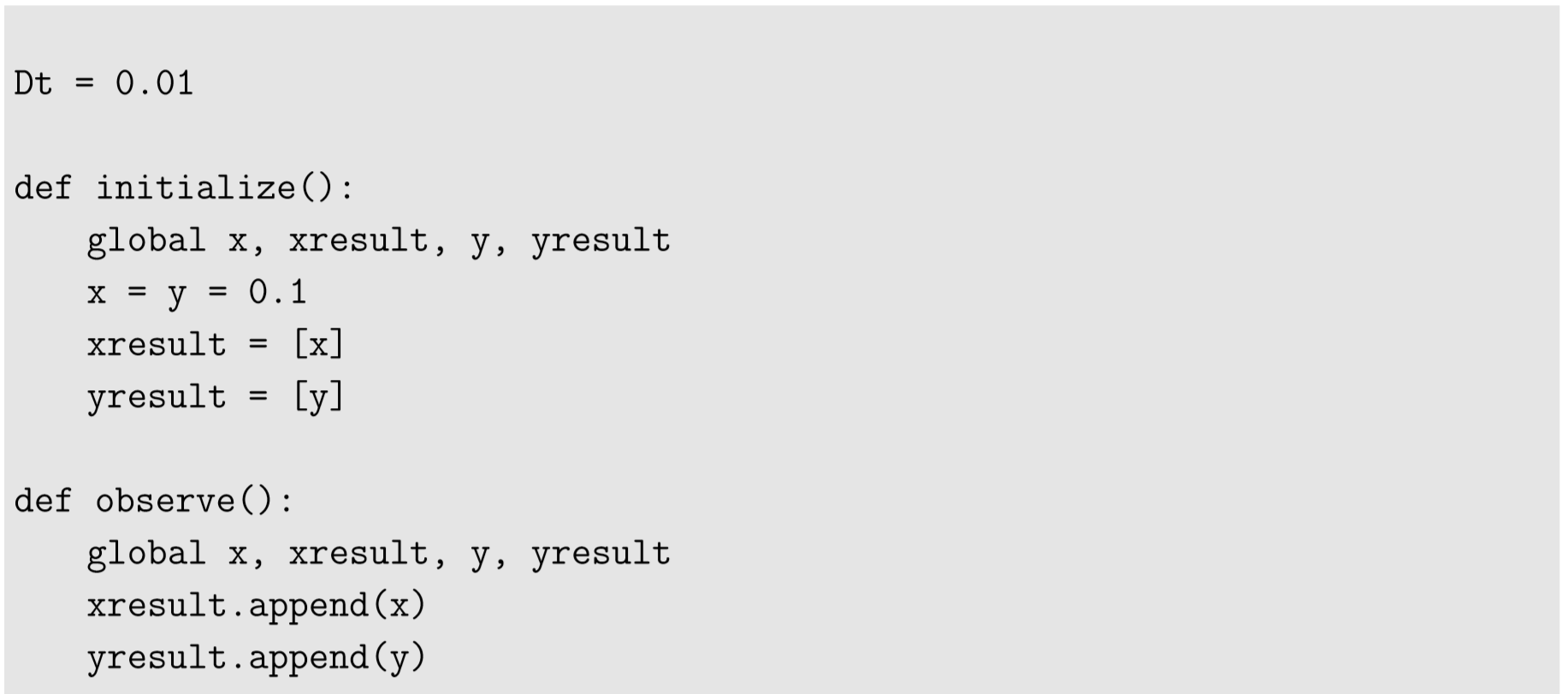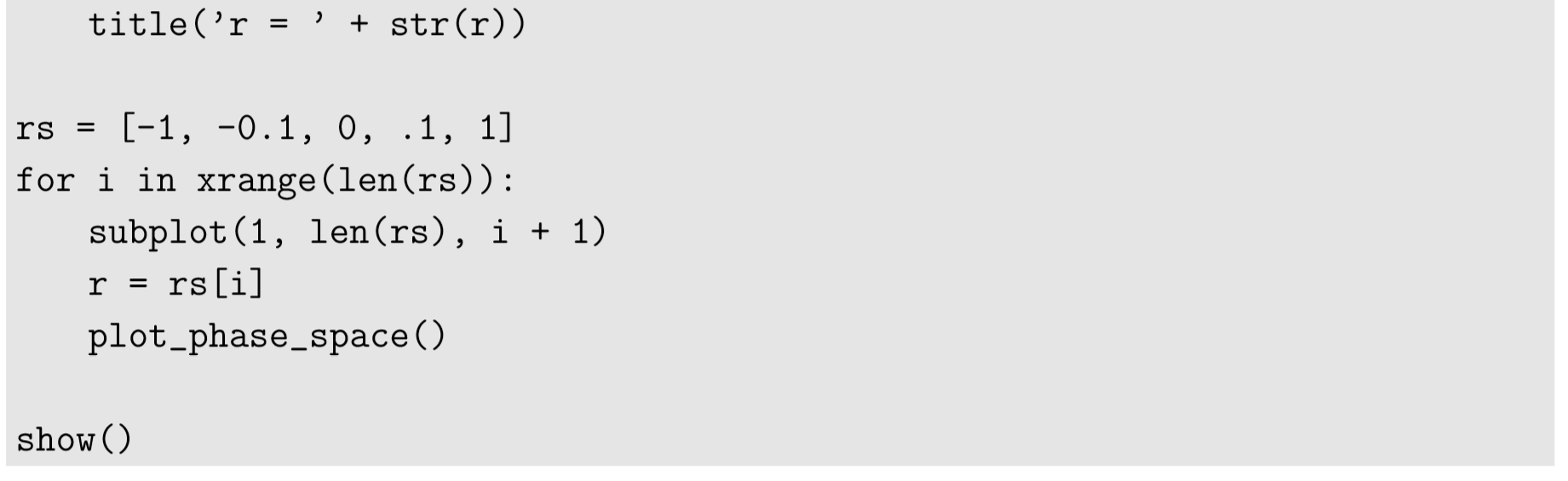$$\newcommand{\id}{\mathrm{id}}$$ $$\newcommand{\Span}{\mathrm{span}}$$ $$\newcommand{\kernel}{\mathrm{null}\,}$$ $$\newcommand{\range}{\mathrm{range}\,}$$ $$\newcommand{\RealPart}{\mathrm{Re}}$$ $$\newcommand{\ImaginaryPart}{\mathrm{Im}}$$ $$\newcommand{\Argument}{\mathrm{Arg}}$$ $$\newcommand{\norm}{\| #1 \|}$$ $$\newcommand{\inner}{\langle #1, #2 \rangle}$$ $$\newcommand{\Span}{\mathrm{span}}$$

# 8.3: Hopf Bifurcations in 2-D Continuous-Time Models

$$\newcommand{\vecs}{\overset { \rightharpoonup} {\mathbf{#1}} }$$ $$\newcommand{\vecd}{\overset{-\!-\!\rightharpoonup}{\vphantom{a}\smash {#1}}}$$$$\newcommand{\id}{\mathrm{id}}$$ $$\newcommand{\Span}{\mathrm{span}}$$ $$\newcommand{\kernel}{\mathrm{null}\,}$$ $$\newcommand{\range}{\mathrm{range}\,}$$ $$\newcommand{\RealPart}{\mathrm{Re}}$$ $$\newcommand{\ImaginaryPart}{\mathrm{Im}}$$ $$\newcommand{\Argument}{\mathrm{Arg}}$$ $$\newcommand{\norm}{\| #1 \|}$$ $$\newcommand{\inner}{\langle #1, #2 \rangle}$$ $$\newcommand{\Span}{\mathrm{span}}$$ $$\newcommand{\id}{\mathrm{id}}$$ $$\newcommand{\Span}{\mathrm{span}}$$ $$\newcommand{\kernel}{\mathrm{null}\,}$$ $$\newcommand{\range}{\mathrm{range}\,}$$ $$\newcommand{\RealPart}{\mathrm{Re}}$$ $$\newcommand{\ImaginaryPart}{\mathrm{Im}}$$ $$\newcommand{\Argument}{\mathrm{Arg}}$$ $$\newcommand{\norm}{\| #1 \|}$$ $$\newcommand{\inner}{\langle #1, #2 \rangle}$$ $$\newcommand{\Span}{\mathrm{span}}$$

For dynamical systems with two or more variables, the dominant eigenvalues of the Jacobian matrix at an equilibrium point could be complex conjugates. If such an equilibrium point, showing an oscillatory behavior around it, switches its stability, the resulting bifurcation is called a Hopf bifurcation. A Hopf bifurcation typically causes the appearance (or disappearance) of a limit cycle around the equilibrium point. A limit cycle is a cyclic, closed trajectory in the phase space that is deﬁned as an asymptotic limit of other oscillatory trajectories nearby. You can check whether the bifurcation is Hopf or not by looking at the imaginary components of the dominant eigenvalues whose real parts are at a critical value (zero); if there are non-zero imaginary components, it must be a Hopf bifurcation.

Here is an example, a dynamical model of a nonlinear oscillator, called the van der Pol oscillator:

$\dfrac{d^{2}x}{xt^{2}} +r(x^{2}-1)\dfrac{dx}{dt} +x=0 \label{(8.20)}$

This is a second-order differential equation, so we should introduce an additional variable $$y = dx/dt$$ to make it into a 2-D ﬁrst-order system, as follows:

\begin{align} \dfrac{dx}{dt} =y \label{(8.21)} \\[4pt] \dfrac{dy}{dt} &=r(x^{2}-1)y-x \label{(8.22)} \end{align}

From these, we can easily show that the origin, $$(x,y) = (0,0)$$, is the only equilibrium point of this system. The Jacobian matrix of this system at the origin is given as follows:

$\begin {pmatrix} 0 & 1 \\ 2rxy-1 & -r(x^{2}-1) \end {pmatrix}\ |_{(x,y)=(0,0) =}\begin{pmatrix}0 & 1 \\-1 & r\end{pmatrix} \label{(8.23)}$

The eigenvalues of this matrix can be calculated as follows:

$\begin {vmatrix} 0-\lambda & 1 \\-1 & r-\lambda\end {vmatrix} =0 \label{(8.24)}$

$-\lambda(r-\lambda) +1 =\lambda^{2} -r\lambda +1 =0\label{(8.25)}$

$\lambda =\dfrac{r \pm \sqrt{r^{2}-4}}{2} \label{(8.26)}$

The critical condition for a bifurcation to occur is

$Re(\lambda) =0,\label{(8.27)}$

whose left hand side can be further detailed as

$Re(\lambda) =\begin{cases}\dfrac{r \pm \sqrt{r^{2}-4}}{2} & \text{if}\ r^{2}\geq 4, \text{or} \\ \dfrac{r}{2} & \text{if}\ r^{2} <4.\end{cases}\label{(8.28)}$

The ﬁrst case can’t be zero, so the only critical condition for a bifurcation to occur is the second case, i.e.

$$r=0$$, when $$Re(\lambda) =0$$ and $$Im(\lambda) =\pm i$$. $$\label{(8.29)}$$

This is a Hopf bifurcation because the eigenvalues have non-zero imaginary parts when the stability change occurs. We can conﬁrm this analytical prediction by numerical simulations of the model with systematically varied $$r$$, as follows:Figure 8.3.1 shows the results where a clear transition from a stable spiral focus (for $$r < 0)$$ to an unstable spiral focus surrounded by a limit cycle (for $$r > 0)$$ is observed.Figure $$\PageIndex{1}$$: Visual output of Code 8.2.

Exercise $$\PageIndex{1}$$: FitzHugh-Nagumo model

The FitzHugh-Nagumo model [30, 31] is a simpliﬁed model of neuronal dynamics that can demonstrate both $$excited$$ and $$resting$$ behaviors of neurons. In a normal setting, this system’s state converges and stays at a stable equilibrium point (resting), but when perturbed, the system’s state moves through a large cyclic trajectory in the phase space before coming back to the resting state, which is observed as a big pulse when plotted over time (excitation). Moreover, under certain conditions, this system can show a nonlinear oscillatory behavior that continuously produces a sequence of pulses. The behavioral shift between convergence to the resting state and generation of a sequence of pulses occurs as a Hopf bifurcation, where the external current is used as a control parameter. Here are the model equations:

$\dfrac{dx}{dt} =c(x-\dfrac{x^{3}}{3}+y+z) \label{(8.30)}$

$\dfrac{dy}{dt} =-\dfrac{x -a +by}{c} \label{(8.31)}$

$$z$$ is the key parameter that represents the external current applied to the neuron. Other parameters are typically constrained as follows:

$1-\dfrac{2}{3}b <a< 1 \label{(8.32)}$

$0<b<1 \label{(8.33)}$

$b<c^{2}\label (8.34)$

With $$a = 0.7$$, $$b = 0.8$$, and $$c = 3$$, do the following:

• Numerically obtain the equilibrium point of this model for several values of $$z$$, ranging between -2 and 0. There is only one real equilibrium point in this system.
• Apply the result obtained above to the Jacobian matrix of the model, and numerically evaluate the stability of that equilibrium point for each value of $$z$$.
• Estimate the critical thresholds of $$z$$ at which a Hopf bifurcation occurs. There are two such critical thresholds.
• Draw a series of its phase spaces with values of $$z$$ varied from 0 to -2 to conﬁrm your analytical prediction.